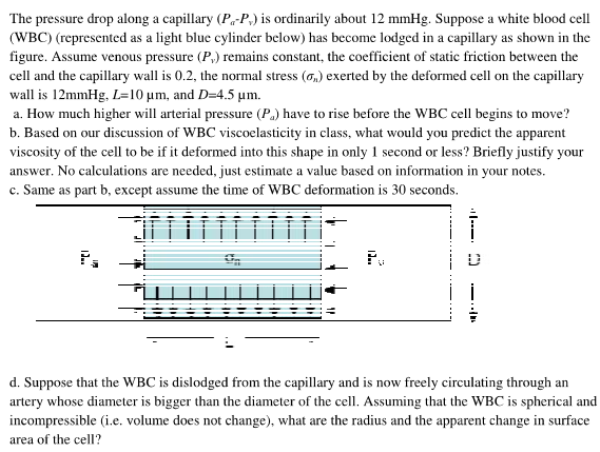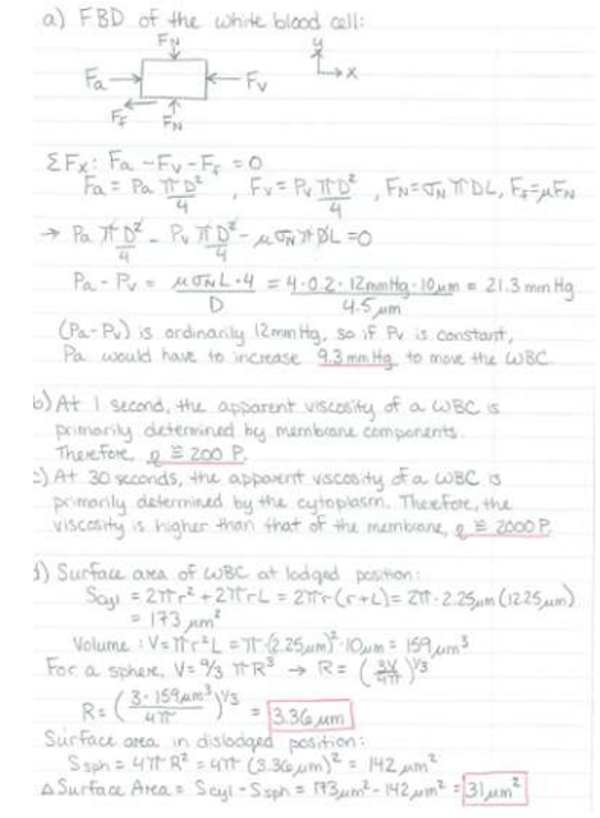The pressure drop along a capillary is ordinarily about 12 mmHg. Suppose a white blood cell (WBC) has become lodged in a capillary as shown in the figure. Assume venous pressure Pv remains constant, the coefficient of static friction between the cell and the capillary wall is 0.2, the normal stress exerted by the deformed cell on the capillary wall is 12 mmHg, L=10 um, and D=4.5 um. a) How much higher will arterial pressure Pa ahve to rise before the WBC cell begins to move? b) Based on our discussion of WBC viscoelasticity in class, what would you predict the apparent viscosity of the cell to be if it deformed into this shape in only 1 second or less? Briefly justify your answer. c) Same as part b, except assume the time of WBC deformation is 30 seconds. d) Suppose that the WBC is dislodged from the capillary and isn ow freely circulating through an artery whose diameter is bigger than the diameter of the cell. Assuming that the WBC is spherical and incompressible, what are the radius and the aparent change in surface area of the cell?The pressure drop along a capillary is ordinarily about 12 mmHg. Suppose a white blood cell (WBC) has become lodged in a capillary as shown in the figure. Assume venous pressure Pv remains constant, the coefficient of static friction between the cell and the capillary wall is 0.2, the normal stress exerted by the deformed cell on the capillary wall is 12 mmHg, L=10 um, and D=4.5 um. a) How much higher will arterial pressure Pa ahve to rise before the WBC cell begins to move? b) Based on our discussion of WBC viscoelasticity in class, what would you predict the apparent viscosity of the cell to be if it deformed into this shape in only 1 second or less? Briefly justify your answer. c) Same as part b, except assume the time of WBC deformation is 30 seconds. d) Suppose that the WBC is dislodged from the capillary and isn ow freely circulating through an artery whose diameter is bigger than the diameter of the cell. Assuming that the WBC is spherical and incompressible, what are the radius and the aparent change in surface area of the cell?

biomechanics page 1 biomechanics biomechanics biomechanics biomechanics biomechanics biomechanics biomechanics biomechanics page 2